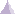# Spring PropertySpring property is a physical property assigned to a section of a part (1D).

The input and output characteristics are:

• Input:
• Translational stiffness
• Rotational stiffness
• Output:
• Point force vector
• Point moment vector

The output characteristics can be expressed at the given positions of the element and in different axis system:

 Position Axis System Characteristics Center of element Nodes of element Gauss point Global Local Point force vectorPoint moment vector